Published: 15 August 2014

# Experimental and numerical studies on multi-spherical sliding friction isolation bearing

Qiang Han1
Jianian Wen2
Liangliang Lin3
Junfeng Jia4
1, 2, 3, 4Key Laboratory of Urban Security and Disaster Engineering of Ministry of Education, Beijing University of Technology, Beijing, 100124, China
1, 2, 3, 4Beijing Collaborative Innovation Center for Metropolitan Transportation, Beijing, 100124, China
Corresponding Author:
Qiang Han
Views 207

#### Abstract

An innovative multi-spherical sliding friction isolation (MSFI) bearing has recently been developed. The novel isolator has efficient energy dissipation capacity and enough displacement capacity under strong earthquake excitations. The MSFI bearing is completely passive devices, yet shows smart stiffness and smart damping under external excitation. The principles of operation and force-displacement relationship of the novel isolator are presented in this paper. The sliding order of all sliding surfaces and force-displacement hysteretic relationship are verified through a displacement-control testing program, and numerical analysis of the MSFI bearing under low cyclic loading is carried out based on ABAQUS program. The results show the sliding order and force-displacement relationship of the MSFI bearing derived from theoretical analysis results and numerical simulation results are well agree with experimental data which the compression-shear testing of the MSFI bearing specimen with the identical curvature radii and friction coefficients. The adaptive behavior of MSFI bearing permits the isolation system to be separately optimized for multiple levels of seismic intensity and ground motions.

## 1. Introduction

There are two modern design approaches intended for reducing destructive effects on bridge structures caused by strong earthquakes. One is used isolation device, which is a strategy that attempts to reduce the seismic forces to near the elastic capacity of the structural member, thereby eliminating or reducing the inelastic deformations. The main concept in isolation is to reduce the fundamental frequency of structural vibration to a value lower than the predominant energy-containing frequencies of the earthquake. The other is used energy dissipation device or increased damping, which is reducing the amount of seismic energy input into the structure. In this way it is possible to limit large plastic deformations caused by the natural period enlargement. This stiffness decreases because of higher elongations due to nonlinear response, decoupling deck from pier under strong seismic events, and then increasing protection efficiency.

Sliding friction isolation bearing is the recently developed seismic isolation devices for buildings and bridges in practice in China and the United States. Sliding friction isolation bearing can be divided into three types: (1) Friction Pendulum System (FPS). The FPS is invented by Zayas  et al. (1990), and the restoring force behavior and test evaluation of the dynamic behavior of this FPS bearing system were carried out by Mokha [2, 3] et al. (1990, 1991) and Tsopelas [4, 5] et al. (1991). (2) Double Concave Friction Pendulum (DCFP). The numerous theoretical studies and laboratory tests are conducted to investigate the behavior of DCFP bearings under dynamic conditions by Tsai [6, 7] et al. (2003, 2005), and force-displacement hysteretic model of the DCFP bearing is presented by Constantinou [8, 9] et al. (2004, 2006). (3) Triple Friction Pendulum Bearing (TFPB). The lateral force-displacement model and behavior of the TFPB bearing are described by Fenz [11-13] et al. (2008) and Fadi  et al. (2010), and Deng  et al. (2011).

The main objectives of this paper are: 1) to expound the principles of operation and force-displacement relationship of the MSFI bearing; 2) to provide theoretical analysis and numerical simulation method of sliding order of all sliding surfaces and force-displacement hysteretic relationship; 3) to verify sliding order and the hysteretic model of MSFI bearing given in this paper are reasonable and accurate by compression-shear testing of the MSFI bearing.

## 2. Configuration of MSFI bearing

The cross section and its parameters of MSFI bearing are shown in Fig. 1 and Table 1, respectively. The MSFI bearing consists of four concave stainless steel sliding surfaces and three independent isolator systems which are separated by an internal cylindrical slider.

Table 1Nomenclature

 Parameter Description Parameter Description $i$ the $i$th sliding surface ${\alpha }_{i}$ the rotation angle of each slider ${R}_{i}$ the radius of sliding surface ${\mu }_{i}$ the friction coefficient of each sliding surface ${h}_{i}$ the radial distance between the $i$th spherical surface and the pivot point $F$ the lateral force ${R}_{ei}$ the effective radius of sliding surface, ${R}_{ei}={R}_{i}-{h}_{i}$ $P$ the vertical load ${d}_{i}$ the actual displacement of each slider ${N}_{i}$ the normal force on each sliding surface ${D}_{i}$ the displacement capacity of each slider ${f}_{i}$ the friction force on each sliding surface ${F}_{si}$ the horizontal restriction force ${F}_{ri}$ the additional force on the slider

The inner concave sliders incorporate sliding surfaces (having radius of ${R}_{e2}$ and ${R}_{e3}$, where ${R}_{e2}={R}_{e3}<<{R}_{e1}={R}_{e4}$) and steel-PTFE interfaces (having coefficients ${\mu }_{2}$ and ${\mu }_{3}$, where ${\mu }_{4}\ge {\mu }_{1}\ge {\mu }_{2}={\mu }_{3}$). Similarly, the outer concave sliders consist of sliding surfaces (having radius of ${R}_{e1}$ and ${R}_{e4}$) and steel-PTFE interfaces (having coefficients ${\mu }_{1}$ and ${\mu }_{4}$). Due to the changeable stiffness and damping at different sliding stages, the isolator structures with MSFI bearing have the ability to undergo multiple levels of external excitations.

Fig. 1Multi-spherical sliding friction isolation bearing: a) photo of isolation bearing and b) cross section of MSFI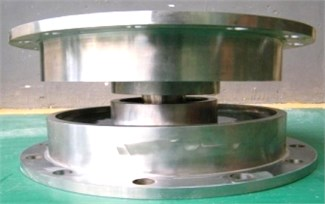a)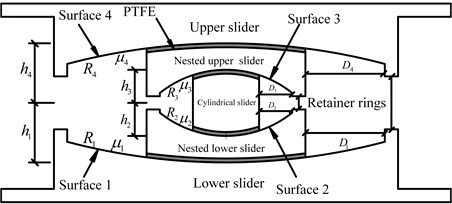b)

## 3. Force-displacement relationship of MSFI bearing

### 3.1. The MSFI bearing with different friction coefficient

From the rest, the bearing could have multiple sliding stages with the increasing of lateral force $F\text{,}$ which are determined by the equilibrium formula on each sliding surface [11-15]. The analytical diagrams of MSFI bearing at different sliding stages are shown in Fig. 2. This diagrams presents the force and displacement relationship for the MSFI bearing at each regime . It is assumed that (a) ${R}_{e2}={R}_{e3}<<{R}_{e1}={R}_{e4}$, (b) ${\mu }_{4}\ge {\mu }_{1}\ge {\mu }_{2}={\mu }_{3}$ in this paper.

Fig. 2Analytical diagrams of MSFI bearing: a) displaced shape and b) free body diagram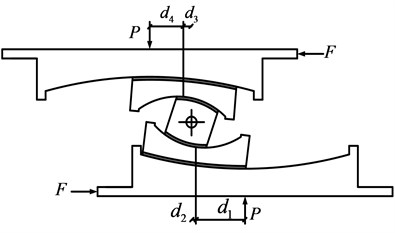a)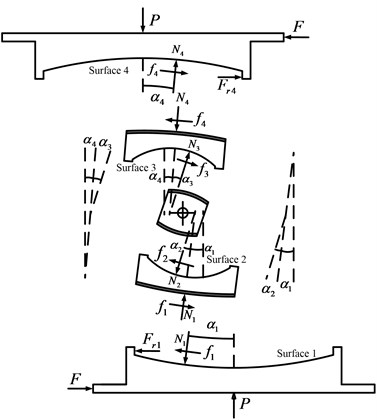b)

### 3.1.1. Sliding regime I

Sliding regime I consist of sliding on surfaces 2 and 3, meanwhile without sliding on surfaces 1 and 4. According to Fig. 2(b), the following relationships are obtained in vertical and horizontal directions:

1
${N}_{1}+{f}_{2}\mathrm{s}\mathrm{i}\mathrm{n}{\alpha }_{2}-{N}_{2}\mathrm{c}\mathrm{o}\mathrm{s}{\alpha }_{2}=0,$
2
${f}_{2}\mathrm{c}\mathrm{o}\mathrm{s}{\alpha }_{2}+{N}_{2}\mathrm{s}\mathrm{i}\mathrm{n}{\alpha }_{2}-{f}_{1}=0,$

and $P={N}_{1}$, $F={f}_{1}$.

Combining Eqs. (1) and (2) and assuming that the relative displacement ${d}_{2}$ is sufficiently small compared with the effective radius ${R}_{e2}$:

3
$F=\frac{P}{{R}_{e2}}{d}_{2}+{f}_{2}.$

Considering the similar analysis on the surface 3:

4
$F=\frac{P}{{R}_{e3}}{d}_{3}+{f}_{3}.$

The force versus total displacement relationship for the bearing during sliding regime I is determined by combining Eqs. (3) and (4):

5
$F=\frac{P}{{R}_{e2}+{R}_{e3}}d+\frac{{f}_{2}{R}_{e2}+{f}_{3}{R}_{e3}}{{R}_{e2}+{R}_{e3}}.$

### 3.1.2. Sliding regime II

When $F={f}_{1}$, motion begins on surface 1, marking the start of sliding regime II. The sliding is a combination of surface 1 and surface 3. The transition occurs at displacement ${d}_{12}$ given by:

6
${d}_{12}=\left({\mu }_{1}-{\mu }_{2}\right){R}_{e2}+\left({\mu }_{1}-{\mu }_{3}\right){R}_{e3}.$

From lower bearing plate of Fig. 2, the relationship governing motion on surface 1 is given:

7
$F=\frac{P}{{R}_{e1}}{d}_{1}+{f}_{1}.$

As reflected in the equations of equilibrium from nested lower slider of Fig. 2:

8
${N}_{1}\mathrm{c}\mathrm{o}\mathrm{s}{\alpha }_{1}+{f}_{2}\mathrm{s}\mathrm{i}\mathrm{n}\left({\alpha }_{1}+{\alpha }_{2}\right)-{N}_{2}\mathrm{c}\mathrm{o}\mathrm{s}\left({\alpha }_{1}+{\alpha }_{2}\right)-{f}_{1}\mathrm{s}\mathrm{i}\mathrm{n}{\alpha }_{1}=0,$
9
${N}_{2}\mathrm{s}\mathrm{i}\mathrm{n}\left({\alpha }_{1}+{\alpha }_{2}\right)+{f}_{2}\mathrm{c}\mathrm{o}\mathrm{s}\left({\alpha }_{1}+{\alpha }_{2}\right)-{N}_{1}\mathrm{s}\mathrm{i}\mathrm{n}{\alpha }_{1}-{f}_{1}\mathrm{c}\mathrm{o}\mathrm{s}{\alpha }_{1}=0.$

Combining Eqs. (8) and (9), for surface 2:

10
$F=P\left(\frac{{d}_{1}}{{R}_{e1}}+\frac{{d}_{2}}{{R}_{e2}}\right)+{f}_{2}.$

Substituting Eq. (7) for Eq. (10):

11
${d}_{2}=\left({\mu }_{1}-{\mu }_{2}\right){R}_{e2}.$

The force versus total displacement relationship for sliding regime II is:

12
$F=\frac{P}{{R}_{e1}+{R}_{e3}}d+\frac{{f}_{1}\left({R}_{e1}-{R}_{e2}\right)+{f}_{2}{R}_{e2}+{f}_{3}{R}_{e3}}{{R}_{e1}+{R}_{e3}}.$

### 3.1.3. Sliding regime III

Sliding will initiate on surface 4 when $F$ equals ${f}_{4}$, and it stops on surface 3. The sliding is a combination of surfaces 1 and 4. The transition occurs at displacement ${d}_{23}$ given by:

13
${d}_{23}={d}_{12}+\left({\mu }_{4}-{\mu }_{1}\right)\left({R}_{e1}+{R}_{e3}\right).$

The relative displacements ${d}_{3}$ and ${d}_{4}$:

14
${d}_{3}={R}_{e3}\mathrm{s}\mathrm{i}\mathrm{n}{\alpha }_{3},$
15
${d}_{4}={R}_{e4}\mathrm{s}\mathrm{i}\mathrm{n}{\alpha }_{4}.$

Motion on surface 1 is still governed by Eq. (12) and motion on surface 2 is still governed by Eq. (15). Similarly, it follows that for surface 4 and for surface 3:

16
$F=\frac{P}{{R}_{e4}}{d}_{4}+{f}_{4},$
17
$F=P\left(\frac{{d}_{3}}{{R}_{e3}}+\frac{{d}_{4}}{{R}_{e4}}\right)+{f}_{3}.$

The force versus total displacement relationship for sliding regime III based on Eq. (7), (10), (16) and (17) is:

18
$F=\frac{P}{{R}_{e1}+{R}_{e4}}d+\frac{{f}_{1}\left({R}_{e1}-{R}_{e2}\right)+{f}_{2}{R}_{e2}+{f}_{3}{R}_{e3}+{f}_{4}\left({R}_{e4}-{R}_{e3}\right)}{{R}_{e1}+{R}_{e4}}.$

### 3.1.4. Sliding regime IV

Sliding regime IV begins, when contact is made with the displacement restrainer on surface 1 and sliding changes from surfaces 1 and 4 to surfaces 2 and 4. The displacement on surface 1 is ${d}_{1}={D}_{1}$, and the lateral force, ${F}_{s1}$ is:

19
${F}_{s1}=\frac{P}{{R}_{e1}}{D}_{1}+{f}_{1}.$

The transition between sliding regimes occurs at total displacement of ${d}_{34}$, given by:

20
${d}_{34}={d}_{23}+{D}_{1}\left(1+\frac{{R}_{e3}}{{R}_{e1}}\right)-\left({\mu }_{4}-{\mu }_{1}\right)\left({R}_{e1}+{R}_{e4}\right).$

In Fig. 2, it is assumed that the displacement restrainer is rigid, the force versus displacement relationship governing motion on surface 1 is:

21
$F=\frac{P}{{R}_{e1}}{D}_{1}+{f}_{1}+{F}_{r1}.$

The force versus displacement relationship governing motion on surface 2 is:

22
$F=P\left(\frac{{D}_{1}}{{R}_{e1}}+\frac{{d}_{2}}{{R}_{e2}}\right)+{f}_{2}.$

Nothing has changed on the upper surfaces so motion on surfaces 3 and 4 is still governed by Eqs. (16) and (17), respectively. Therefore, the force-total displacement relationship is:

23
$F=\frac{P}{{R}_{e2}+{R}_{e4}}\left(d-{d}_{34}\right)+\frac{P}{{R}_{e1}}{D}_{1}+{f}_{1}.$

### 3.1.5. Sliding regime V

Sliding regime 5 begins when contact is made with the displacement restrainer on surface 4. Motion changes from sliding on surfaces 2 and 4 to sliding on surfaces 2 and 3. At the transition point, the relative displacement on surface 4 is ${d}_{4}={D}_{4}$ and the horizontal force, ${F}_{s4}$, is:

24
${F}_{s4}=\frac{P}{{R}_{e4}}{D}_{4}+{f}_{4}.$

The transition between sliding regimes occurs at a total displacement of ${d}_{45}$, given by:

25
${d}_{45}={d}_{34}+\left[\left(\frac{{D}_{4}}{{R}_{e4}}+{\mu }_{4}\right)-\left(\frac{{D}_{1}}{{R}_{e1}}+{\mu }_{1}\right)\right]\left({R}_{e2}+{R}_{e4}\right).$

From similar above analysis, it follows that for surface 4 and for surface 3:

26
$F=\frac{P}{{R}_{e4}}{D}_{4}+{f}_{4}+{F}_{r4},$
27
$F=P\left(\frac{{D}_{4}}{{R}_{e4}}+\frac{{d}_{3}}{{R}_{e3}}\right)+{f}_{3}.$

Motion continues on surface 2 with the slider bearing on the displacement restrainer of surface 1. Combining the force versus displacement relationships gives:

28
$F=\frac{P}{{R}_{e2}+{R}_{e3}}\left(d-{d}_{45}\right)+\frac{P}{{R}_{e4}}{D}_{4}+{f}_{4}.$

Five different sliding regimes are presented in Fig. 3(a).

Fig. 3Hysteretic loops of the bearing a) μ4≥μ1≥μ2=μ3 and b) μ1=μ2=μ3=μ4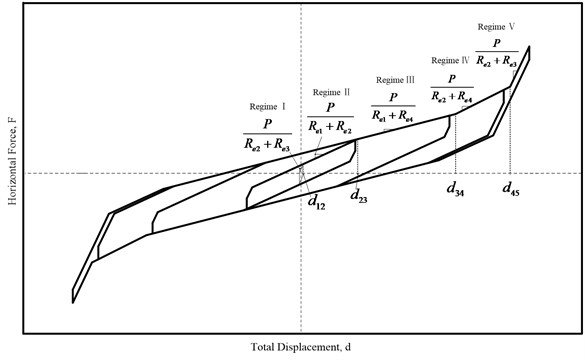a)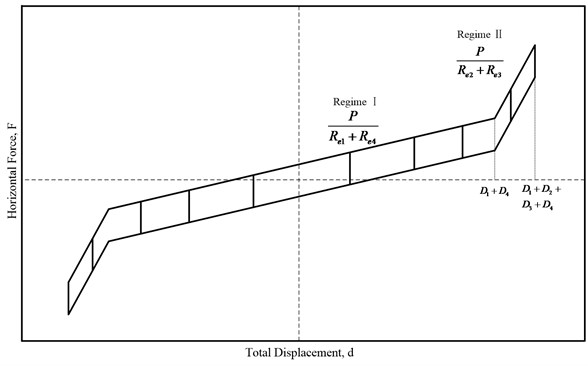b)

### 3.2. The bearing with the equal friction coefficient

Assuming that friction coefficient of a MSFI bearing with ${\mu }_{1}={\mu }_{2}={\mu }_{3}={\mu }_{4}$, the mechanism and sliding order of the bearing will be changed.

From the rest, sliding consists of sliding on surfaces 1 and 4, meanwhile without sliding on surfaces 2 and 3. The MSFI bearing presents a relatively lower stiffness initially. The force versus displacement relationship is given:

29
$F=\frac{P}{{R}_{e1}+{R}_{e4}}d+f,d\le {D}_{1}+{D}_{4}.$

The stiffness will increase with the increase of the displacement amplitude, and the force-displacement relationship is given:

30
$F=\frac{P}{{R}_{e2}+{R}_{e3}}d-\frac{P\left({D}_{1}+{D}_{4}\right)\left({R}_{e1}+{R}_{e4}-{R}_{e2}-{R}_{e3}\right)}{\left({R}_{e1}+{R}_{e4}\right)\left({R}_{e2}+{R}_{e3}\right)}+f,$
${D}_{1}+{D}_{4}

Two different sliding regimes are presented in Fig. 3(b). The force-displacement formulas are simplified, when the coefficients on the all surfaces are same.

### 3.3. Discussion about the hysteretic behavior

The hysteretic loop reflects the energy dissipation and the stiffness properties of the isolation bearing, and then the hysteresis model of the isolation bearings can be used to analyze seismic response of the structures under earthquake excitation. The traditional FP bearing is an isolator which is bilinear hysteresis system with the unchangeable stiffness and damping. As for the developed MSFI bearing in this paper, it is a modified FP bearing with smart hysteresis behavior which exhibits adaptive changes in stiffness and damping at different sliding regimes (as shown in Fig. 3).

## 4. 3D FE analysis of MSFI bearing

### 4.1. 3D FE model

The force versus displacement behavior and sliding order of the MSFI bearing is simulated by adopted ABAQUS program. The FEM model was employed the same effective radius (470 mm) of upper and lower sliders. Additionally, the nested sliders were assembled having the same radius (80 mm). Each surface of the slider was coated with PTFE, and with the thickness of 2 mm. The geometry dimensions of isolation bearing is same in numerical simulation and following experimental studies.

Two assumptions were made in the modeling process:

1) The material properties are in the elastic stage in ABAQUS model.

2) It should be noticed that the friction coefficient between steel and PTFE interface is affected by the contact pressure temperature and other conditions. In this study, the friction coefficient for each sliding surface is considered to be a constant in all direction.

The model of bearing adopts eight nodes hexahedron linear reduced integral unit (C3D8R) which is suitable for elastic-plastic analysis and contact analysis . The MSFI bearing material models for this simulation are stainless steel (Young’s modulus 210000 MPa, Poisson’s rate 0.31) and PTFE (Young’s modulus 280 MPa, Poisson’s rate 0.42). The smallest friction coefficients are those of the nested slider surfaces. The friction properties are characterized by: 1) ${\mu }_{4}=\text{0.1}$, ${\mu }_{1}=\text{0.05}$, ${\mu }_{2}={\mu }_{3}=\text{0.01}$, 2) ${\mu }_{1}={\mu }_{2}={\mu }_{3}={\mu }_{4}=\text{0.04}$.

The bearing FEM model is subjected to a sequence of load steps. In step 1, the bearing was subjected to a concentrated force of 100 kN. In step 2, the horizontal motion was displacement-controlled which was imposed a sinusoidal horizontal displacement of $D=A\mathrm{s}\mathrm{i}\mathrm{n}\left(2\pi ft\right)$, with $f=$0.5 Hz, $A=$5, 50, 100, 120, 150 mm, separately.

### 4.2. Numerical simulation results

The numerical simulation results obtained from the ABAQUS model described above will be compared with the theoretical analysis results. Fig. 4 shows the operation mechanism of MSFI bearing with different friction coefficients as the increasing of the amplitude of displacement:

1. The sliding initiates on surface 2 and 3, without sliding on the other sliding surfaces (Fig. 4(b)).

2. In sliding regime II, sliding is stopped on surface 2 and initiates on surface 1 and 3 (Fig. 4(c)).

3. In sliding regime III, sliding is stopped on surface 3 and initiates on surface 1 and 4 (Fig. 4(d)).

4. In sliding regime IV, slider contacts restrainer on surface 1 and sliding on surface 2 and 4 (Fig. 4(e)).

5. In sliding regime V, slider contacts restrainer on surface 4 and sliding on surface 2 and 3 (Fig. 4(f)).

Fig. 4Sliding mechanisms of MSFI bearing with different coefficient of friction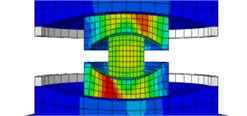a) Sliding from rest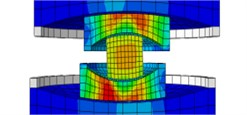b) Regime I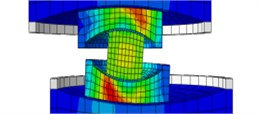c) Regime II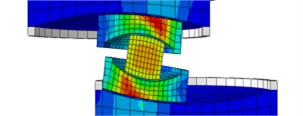d) Regime III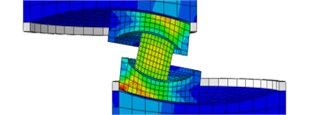e) Regime IV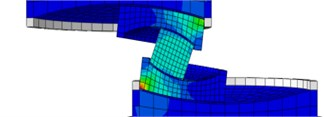f) Regime V

Fig. 5 shows the operation mechanism of MSFI bearing with equal friction coefficients as the increasing of the amplitude of displacement:

1. The sliding initiates on surface 1 and 4, without sliding on the other sliding surfaces (Fig. 5(b)).

2. In sliding regime II, slider contacts restrainer on surface 1, 4 and sliding on surface 2 and 3 (Fig. 5(c)).

Fig. 5Sliding mechanisms of MSFI bearing with equal coefficient of friction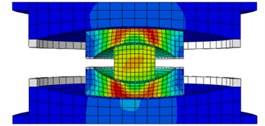a) Sliding from rest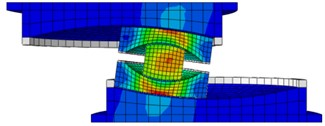b) Regime I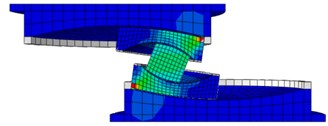c) Regime II

It can be seen that the sliding mechanisms of ABAQUS simulation are good agreement with theoretical analysis in different sliding regimes.

Fig. 6 shows the force and displacement hysteresis loops using ABAQUS program compared with the theoretical analysis. Generally speaking, there is a good agreement between numerical simulation results using the ABAQUS program and the theoretical analytical results. The major discrepancy between analytical results and simulation data is that the analytical model underestimates the horizontal force when the amplitude of displacement is increasing. Moreover, it should be noted that the transition points between stiffness shown in Fig. 5(a) are slightly different for equal friction coefficient. The reason that the theory assumes that $\alpha$ (rotation angle) is much small, whereas the influence of $\alpha$ can’t be neglected as the increasing of the amplitude.

Fig. 6Hysteretic loops of multi-spherical sliding friction isolation bearing: a) different friction coefficient and b) equal friction coefficient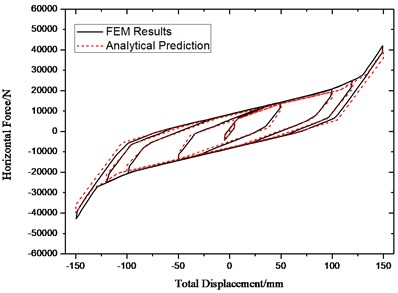a)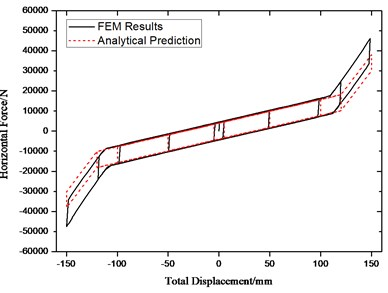b)

## 5. Experimental verification

### 5.1. Testing setup and procedure

The test was conducted at the Key Laboratory of Urban Security and Disaster Engineering of Ministry of Education in China. At low velocity, the coefficient of friction is highly dependent on velocity as they are related by a negative exponential relationship (seen as Fig. 7). The relative differences are substantial, but there is still small absolute difference in the range of values compared with high speed . The test was conducted at low velocity (only 1 mm/s), so that the friction coefficients can be controlled ${\mu }_{1}={\mu }_{2}={\mu }_{3}={\mu }_{4}=\text{0.04}$ through smearing silicone grease over the stainless-PTFE interfaces.

Fig. 7Illustration of the range in the coefficient of friction for low speed testing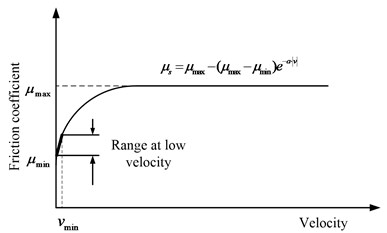The instruments consist of two vertical actuators and one horizontal actuator, as shown in Fig. 8. The vertical actuator was imposed axial loads (100 KN) on the MSFI bearing and the horizontal actuator was imposed to generate the lateral force and expected displacement. Moreover, the horizontal displacement was measured by LVDT. The testing photo of isolation bearing is shown in Fig. 9.

Fig. 8Diagram of setup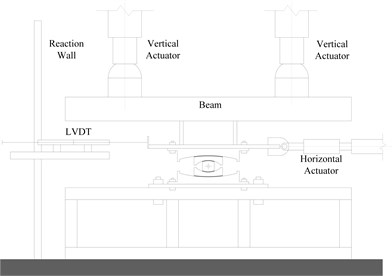Fig. 9Testing photo of the MSFI bearing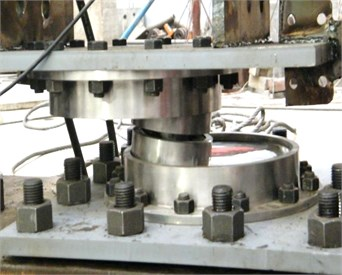### 5.2. Experimental results of the MSFI bearing

The results of numerical simulation and theoretical analysis were compared with the hysteretic curves obtained from the experimental data. In general, the experimental data are well agree with the theoretical results and the simulation results with the friction coefficient of MSFI bearing, as shown in Fig. 10. Fig. 10 also depicts the major discrepancy between theory and experiment occurs at more than the amplitude of 100 mm, where the transition points between stiffness is slightly earlier on surface 4. This is not obvious in the mathematical model. It can be seen that there is a 10 %-20 % deviations in terms of maximum displacement between the simulation and experiment results. The reason is that the greater error in the small angle assumption in theoretical analysis and the effect of overturning moment in the testing. So the influence of errors introduced at large displacements can’t be neglected and overturning moment should be considered in seismic analysis of buildings and bridges with MSFI bearings. Generally, the mathematical model of MSFI bearing presented in this paper is reasonable and accurate, which can be utilized to predict dynamic response of the hysteresis behavior of MSFI bearings.

Fig. 10Comparison of analytical, simulation and experimental results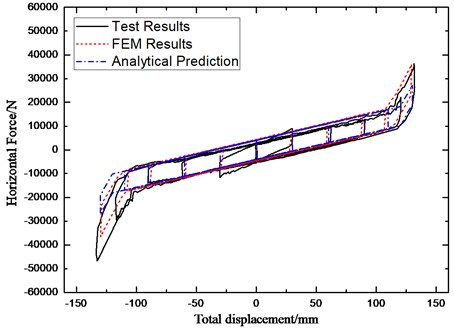## 6. Conclusions

The novel MSFI bearing has recently been developed, and the principles of operation and force-displacement relationship of the novel isolator are presented in this study. The results can be concluded as follow:

1) The novel isolator has efficient energy dissipation capacity and enough displacement capacity under strong earthquake excitations. The MSFI bearing is completely passive devices, yet shows smart stiffness and smart damping under external excitation, which adapts for performance-based seismic bridge design for multiple levels of ground motions.

2) The sliding mechanisms of MSFI bearing using ABAQUS program are good agreement with theoretical analysis in different sliding regimes, and this sliding mechanism give a full process of the MSFI bearing movement under different levels of external excitation.

3) The mathematical model of MSFI bearing presented in this paper is reasonable and accurate, which can be utilized to predict dynamic response of the hysteresis behavior of MSFI bearings.

16 April 2014
Accepted
19 June 2014
Published
15 August 2014
Keywords
seismic isolation
friction isolation bearing
restoring force
smart behavior
bearing testing
Acknowledgements

This research is jointly funded by Beijing Natural Science Foundation (No. 8122003), the National Natural Science Fund of China (NSFC) (Grants No. 51178008), the research project of Beijing Municipal Commission of Education (Grant KZ201410005011) and the National Program on Key Basic Research Project (Grant No. 2011CB013600). Their supports are gratefully acknowledged.